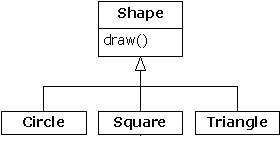# 11.1 对RTTI的需要``````//: Shapes.java
package c11;
import java.util.*;

interface Shape {
void draw();
}

class Circle implements Shape {
public void draw() {
System.out.println("Circle.draw()");
}
}

class Square implements Shape {
public void draw() {
System.out.println("Square.draw()");
}
}

class Triangle implements Shape {
public void draw() {
System.out.println("Triangle.draw()");
}
}

public class Shapes {
public static void main(String[] args) {
Vector s = new Vector();
Enumeration e = s.elements();
while(e.hasMoreElements())
((Shape)e.nextElement()).draw();
}
} ///:~
``````

11.1.1 Class对象

``````//: SweetShop.java
// Examination of the way the class loader works

class Candy {
static {
}
}

class Gum {
static {
}
}

static {
}
}

public class SweetShop {
public static void main(String[] args) {
System.out.println("inside main");
new Candy();
System.out.println("After creating Candy");
try {
Class.forName("Gum");
} catch(ClassNotFoundException e) {
e.printStackTrace();
}
System.out.println(
"After Class.forName(\"Gum\")");
}
} ///:~
``````

``````Class.forName("Gum");
``````

``````inside main
After creating Candy
After Class.forName("Gum")
``````

``````Loading Candy
inside main
After creating Candy
After Class.forName("Gum")
``````

1. 类标记

……等价于……

``````... is equivalent to ...
boolean.class

Boolean.TYPE

char.class

Character.TYPE

byte.class

Byte.TYPE

short.class

Short.TYPE

int.class

Integer.TYPE

long.class

Long.TYPE

float.class

Float.TYPE

double.class

Double.TYPE

void.class

Void.TYPE
``````

11.1.2 造型前的检查

(1) 经典造型，如"(Shape)"，它用RTTI确保造型的正确性，并在遇到一个失败的造型后产生一个ClassCastException违例。

(2) 代表对象类型的Class对象。可查询Class对象，获取有用的运行期资料。

RTTI在Java中存在三种形式。关键字instanceof告诉我们对象是不是一个特定类型的实例（Instance即“实例”）。它会返回一个布尔值，以便以问题的形式使用，就象下面这样：

``````if(x instanceof Dog)
((Dog)x).bark();
``````

``````//: PetCount.java
// Using instanceof
package c11.petcount;
import java.util.*;

class Pet {}
class Dog extends Pet {}
class Pug extends Dog {}
class Cat extends Pet {}
class Rodent extends Pet {}
class Gerbil extends Rodent {}
class Hamster extends Rodent {}

class Counter { int i; }

public class PetCount {
static String[] typenames = {
"Pet", "Dog", "Pug", "Cat",
"Rodent", "Gerbil", "Hamster",
};
public static void main(String[] args) {
Vector pets = new Vector();
try {
Class[] petTypes = {
Class.forName("c11.petcount.Dog"),
Class.forName("c11.petcount.Pug"),
Class.forName("c11.petcount.Cat"),
Class.forName("c11.petcount.Rodent"),
Class.forName("c11.petcount.Gerbil"),
Class.forName("c11.petcount.Hamster"),
};
for(int i = 0; i < 15; i++)
petTypes[
(int)(Math.random()*petTypes.length)]
.newInstance());
} catch(InstantiationException e) {}
catch(IllegalAccessException e) {}
catch(ClassNotFoundException e) {}
Hashtable h = new Hashtable();
for(int i = 0; i < typenames.length; i++)
h.put(typenames[i], new Counter());
for(int i = 0; i < pets.size(); i++) {
Object o = pets.elementAt(i);
if(o instanceof Pet)
((Counter)h.get("Pet")).i++;
if(o instanceof Dog)
((Counter)h.get("Dog")).i++;
if(o instanceof Pug)
((Counter)h.get("Pug")).i++;
if(o instanceof Cat)
((Counter)h.get("Cat")).i++;
if(o instanceof Rodent)
((Counter)h.get("Rodent")).i++;
if(o instanceof Gerbil)
((Counter)h.get("Gerbil")).i++;
if(o instanceof Hamster)
((Counter)h.get("Hamster")).i++;
}
for(int i = 0; i < pets.size(); i++)
System.out.println(
pets.elementAt(i).getClass().toString());
for(int i = 0; i < typenames.length; i++)
System.out.println(
typenames[i] + " quantity: " +
((Counter)h.get(typenames[i])).i);
}
} ///:~
``````

1. 使用类标记

PetCount.java示例可用Java 1.1的类标记重写一遍。得到的结果显得更加明确易懂：

``````//: PetCount2.java
// Using Java 1.1 class literals
package c11.petcount2;
import java.util.*;

class Pet {}
class Dog extends Pet {}
class Pug extends Dog {}
class Cat extends Pet {}
class Rodent extends Pet {}
class Gerbil extends Rodent {}
class Hamster extends Rodent {}

class Counter { int i; }

public class PetCount2 {
public static void main(String[] args) {
Vector pets = new Vector();
Class[] petTypes = {
// Class literals work in Java 1.1+ only:
Pet.class,
Dog.class,
Pug.class,
Cat.class,
Rodent.class,
Gerbil.class,
Hamster.class,
};
try {
for(int i = 0; i < 15; i++) {
// Offset by one to eliminate Pet.class:
int rnd = 1 + (int)(
Math.random() * (petTypes.length - 1));
petTypes[rnd].newInstance());
}
} catch(InstantiationException e) {}
catch(IllegalAccessException e) {}
Hashtable h = new Hashtable();
for(int i = 0; i < petTypes.length; i++)
h.put(petTypes[i].toString(),
new Counter());
for(int i = 0; i < pets.size(); i++) {
Object o = pets.elementAt(i);
if(o instanceof Pet)
((Counter)h.get(
"class c11.petcount2.Pet")).i++;
if(o instanceof Dog)
((Counter)h.get(
"class c11.petcount2.Dog")).i++;
if(o instanceof Pug)
((Counter)h.get(
"class c11.petcount2.Pug")).i++;
if(o instanceof Cat)
((Counter)h.get(
"class c11.petcount2.Cat")).i++;
if(o instanceof Rodent)
((Counter)h.get(
"class c11.petcount2.Rodent")).i++;
if(o instanceof Gerbil)
((Counter)h.get(
"class c11.petcount2.Gerbil")).i++;
if(o instanceof Hamster)
((Counter)h.get(
"class c11.petcount2.Hamster")).i++;
}
for(int i = 0; i < pets.size(); i++)
System.out.println(
pets.elementAt(i).getClass().toString());
Enumeration keys = h.keys();
while(keys.hasMoreElements()) {
String nm = (String)keys.nextElement();
Counter cnt = (Counter)h.get(nm);
System.out.println(
nm.substring(nm.lastIndexOf('.') + 1) +
" quantity: " + cnt.i);
}
}
} ///:~
``````

Pet动态创建好以后，可以看到随机数字已得到了限制，位于1和petTypes.length之间，而且不包括零。那是由于零代表的是Pet.class，而且一个普通的Pet对象可能不会有人感兴趣。然而，由于Pet.class是petTypes的一部分，所以所有Pet（宠物）都会算入计数中。

1. 动态的instanceof

Java 1.1为Class类添加了isInstance方法。利用它可以动态调用instanceof运算符。而在Java 1.0中，只能静态地调用它（就象前面指出的那样）。因此，所有那些烦人的instanceof语句都可以从PetCount例子中删去了。如下所示：

``````//: PetCount3.java
// Using Java 1.1 isInstance()
package c11.petcount3;
import java.util.*;

class Pet {}
class Dog extends Pet {}
class Pug extends Dog {}
class Cat extends Pet {}
class Rodent extends Pet {}
class Gerbil extends Rodent {}
class Hamster extends Rodent {}

class Counter { int i; }

public class PetCount3 {
public static void main(String[] args) {
Vector pets = new Vector();
Class[] petTypes = {
Pet.class,
Dog.class,
Pug.class,
Cat.class,
Rodent.class,
Gerbil.class,
Hamster.class,
};
try {
for(int i = 0; i < 15; i++) {
// Offset by one to eliminate Pet.class:
int rnd = 1 + (int)(
Math.random() * (petTypes.length - 1));
petTypes[rnd].newInstance());
}
} catch(InstantiationException e) {}
catch(IllegalAccessException e) {}
Hashtable h = new Hashtable();
for(int i = 0; i < petTypes.length; i++)
h.put(petTypes[i].toString(),
new Counter());
for(int i = 0; i < pets.size(); i++) {
Object o = pets.elementAt(i);
// Using isInstance to eliminate individual
// instanceof expressions:
for (int j = 0; j < petTypes.length; ++j)
if (petTypes[j].isInstance(o)) {
String key = petTypes[j].toString();
((Counter)h.get(key)).i++;
}
}
for(int i = 0; i < pets.size(); i++)
System.out.println(
pets.elementAt(i).getClass().toString());
Enumeration keys = h.keys();
while(keys.hasMoreElements()) {
String nm = (String)keys.nextElement();
Counter cnt = (Counter)h.get(nm);
System.out.println(
nm.substring(nm.lastIndexOf('.') + 1) +
" quantity: " + cnt.i);
}
}
} ///:~
``````